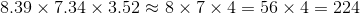# SSAT Upper Level Math : How to estimate

## Example Questions

### Example Question #1 : How To Estimate

Evan wants to tip approximatelyon a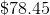restaurant tab. Which of the following comes closest to what he should leave?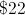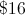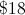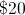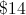Explanation:

The tab can be rounded to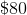is equal to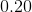.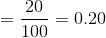Now we can multiply the percent by the total amount.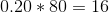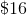is the most reasonable estimate of the recommended tip.

### Example Question #1 : How To Estimate

If the number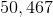is rounded to the nearest hundredth, which of the following expressions would be equal to that value?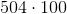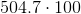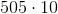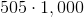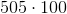Explanation:

Ifis rounded to the nearest hundredth, the result will be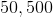Given that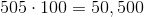, the correct answer is### Example Question #2 : How To Estimate

Estimate the product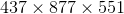by rounding each factor to the nearest hundred, then multiplying.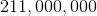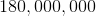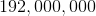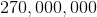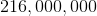Explanation:

437 rounded to the nearest hundred is 400.

877 rounded to the nearest hundred is 900.

551 rounded to the nearest hundred is 600.

Multiply the three whole multiples of 100 to get the desired estimate: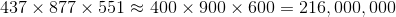### Example Question #2 : Estimation

Estimate the product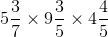by rounding each factor to the nearest unit, then multiplying.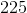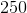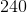Explanation: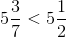, so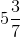rounded to the nearest unit is 5.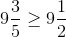, so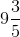rounded to the nearest unit is 10.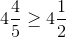, so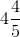rounded to the nearest unit is 5.

Multiply the three whole numbers to get the desired estimate: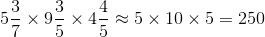### Example Question #3 : How To Estimate

Estimate the result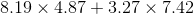by first rounding each number to the nearest unit.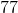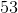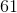Explanation:

8.19 rounded to the nearest unit is 8.

4.87 rounded to the nearest unit is 5

3.27 rounded to the nearest unit is 3.

7.42 rounded to the nearest unit is 7.

The desired estimate can be found as follows: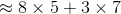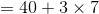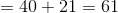### Example Question #3 : How To Estimate

Estimate the result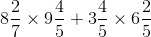by first rounding each number to the nearest unit.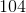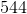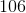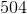Explanation: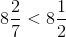, so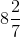rounded to the nearest unit is 8.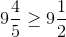, so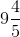rounded to the nearest unit is 10.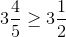, so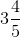rounded to the nearest unit is 4.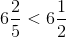, so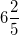rounded to the nearest unit is 6.

The desired estimate can be found as follows: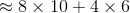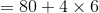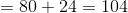### Example Question #2 : How To Estimate

Melissa is trying to come up with a reasonable estimate of the amount she spent on groceries over the last six months. She notices that the six checks she wrote out to the local grocery store are in the following amounts: $187.54,$218.89, $174.74,$104.76, $189.75, and$228.64. By estimating each of the amounts of the checks to the nearest ten dollars, come up with a reasonable estimate for Melissa's total expenditure for groceries.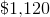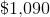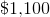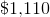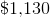Explanation:

Round each of the amounts to the nearest ten dollars as follows:

$187.54 rounds to$190.

$218.89 rounds to$220.

$174.74 rounds to$170.

$104.76 rounds to$100.

$189.75 rounds to$190.

$228.64 rounds to$230.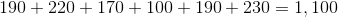### Example Question #4 : How To Estimate

Estimate the product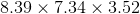by rounding each factor to the nearest unit, then multiplying.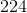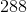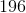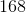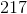Explanation:

8.39 rounded to the nearest unit is 8 because 0.39 is less than 0.5.

7.34 rounded to the nearest unit is 7 because 0.34 is less than 0.5.

3.52 rounded to the nearest unit is 4 because 0.52 is greater than 0.5.

Multiply the three whole numbers to get the desired estimate: